Documentation

### This is machine translation

Mouseover text to see original. Click the button below to return to the English version of the page.

To view all translated materials including this page, select Country from the country navigator on the bottom of this page.

## Bootstrap Aggregation (Bagging) of Regression Trees Using `TreeBagger`

Statistics and Machine Learning Toolbox™ offers two objects that support bootstrap aggregation (bagging) of regression trees: `TreeBagger` created by using `TreeBagger` and `RegressionBaggedEnsemble` created by using `fitrensemble`. See Comparison of TreeBagger and Bagged Ensembles for differences between `TreeBagger` and `RegressionBaggedEnsemble`.

This example shows the workflow for classification using the features in `TreeBagger` only.

Use a database of 1985 car imports with 205 observations, 25 predictors, and 1 response, which is insurance risk rating, or "symboling." The first 15 variables are numeric and the last 10 are categorical. The symboling index takes integer values from -3 to 3.

Load the data set and split it into predictor and response arrays.

```load imports-85 Y = X(:,1); X = X(:,2:end); isCategorical = [zeros(15,1);ones(size(X,2)-15,1)]; % Categorical variable flag```

Because bagging uses randomized data drawings, its exact outcome depends on the initial random seed. To reproduce the results in this example, use the random stream settings.

`rng(1945,'twister')`

### Finding the Optimal Leaf Size

For regression, the general rule is to the set leaf size to 5 and select one third of the input features for decision splits at random. In the following step, verify the optimal leaf size by comparing mean squared errors obtained by regression for various leaf sizes. `oobError` computes MSE versus the number of grown trees. You must set `OOBPred` to `'On'` to obtain out-of-bag predictions later.

```leaf = [5 10 20 50 100]; col = 'rbcmy'; figure for i=1:length(leaf) b = TreeBagger(50,X,Y,'Method','R','OOBPrediction','On',... 'CategoricalPredictors',find(isCategorical == 1),... 'MinLeafSize',leaf(i)); plot(oobError(b),col(i)) hold on end xlabel('Number of Grown Trees') ylabel('Mean Squared Error') legend({'5' '10' '20' '50' '100'},'Location','NorthEast') hold off```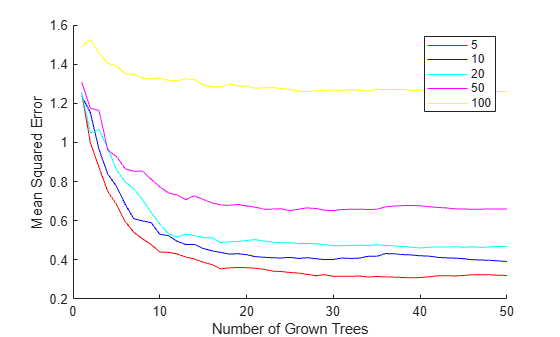The red curve (leaf size 5) yields the lowest MSE values.

### Estimating Feature Importance

In practical applications, you typically grow ensembles with hundreds of trees. For example, the previous code block uses 50 trees for faster processing. Now that you have estimated the optimal leaf size, grow a larger ensemble with 100 trees and use it to estimate feature importance.

```b = TreeBagger(100,X,Y,'Method','R','OOBPredictorImportance','On',... 'CategoricalPredictors',find(isCategorical == 1),... 'MinLeafSize',5);```

Inspect the error curve again to make sure nothing went wrong during training.

```figure plot(oobError(b)) xlabel('Number of Grown Trees') ylabel('Out-of-Bag Mean Squared Error')```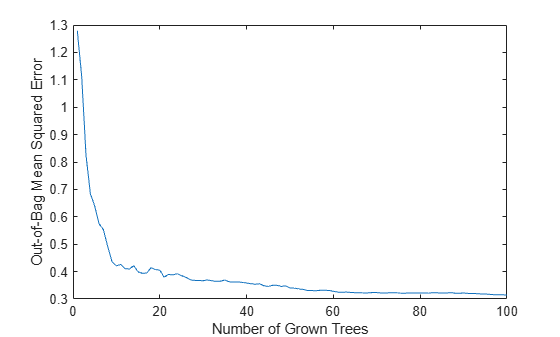Prediction ability should depend more on important features than unimportant features. You can use this idea to measure feature importance.

For each feature, permute the values of this feature across every observation in the data set and measure how much worse the MSE becomes after the permutation. You can repeat this for each feature.

Plot the increase in MSE due to permuting out-of-bag observations across each input variable. The `OOBPermutedPredictorDeltaError` array stores the increase in MSE averaged over all trees in the ensemble and divided by the standard deviation taken over the trees, for each variable. The larger this value, the more important the variable. Imposing an arbitrary cutoff at 0.7, you can select the four most important features.

```figure bar(b.OOBPermutedPredictorDeltaError) xlabel('Feature Number') ylabel('Out-of-Bag Feature Importance')```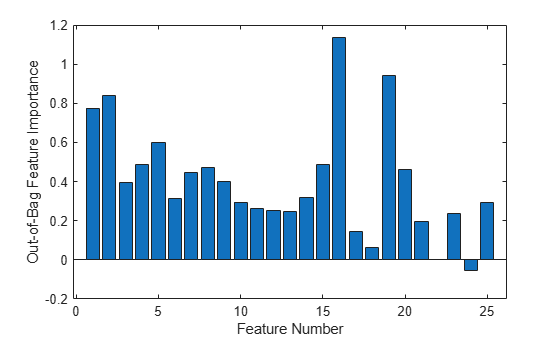`idxvar = find(b.OOBPermutedPredictorDeltaError>0.7)`
```idxvar = 1×4 1 2 16 19 ```
`idxCategorical = find(isCategorical(idxvar)==1);`

The `OOBIndices` property of `TreeBagger` tracks which observations are out of bag for what trees. Using this property, you can monitor the fraction of observations in the training data that are in bag for all trees. The curve starts at approximately 2/3, which is the fraction of unique observations selected by one bootstrap replica, and goes down to 0 at approximately 10 trees.

```finbag = zeros(1,b.NTrees); for t=1:b.NTrees finbag(t) = sum(all(~b.OOBIndices(:,1:t),2)); end finbag = finbag / size(X,1); figure plot(finbag) xlabel('Number of Grown Trees') ylabel('Fraction of In-Bag Observations')```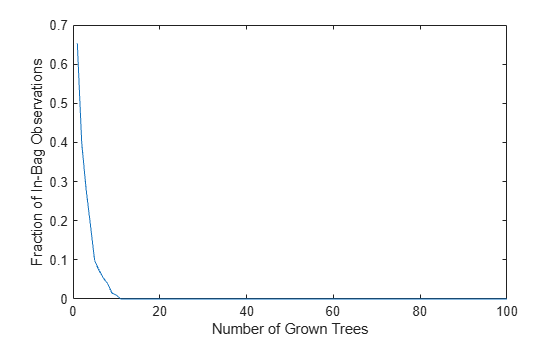### Growing Trees on a Reduced Set of Features

Using just the four most powerful features, determine if it is possible to obtain a similar predictive power. To begin, grow 100 trees on these features only. The first two of the four selected features are numeric and the last two are categorical.

```b5v = TreeBagger(100,X(:,idxvar),Y,'Method','R',... 'OOBPredictorImportance','On','CategoricalPredictors',idxCategorical,... 'MinLeafSize',5); figure plot(oobError(b5v)) xlabel('Number of Grown Trees') ylabel('Out-of-Bag Mean Squared Error')```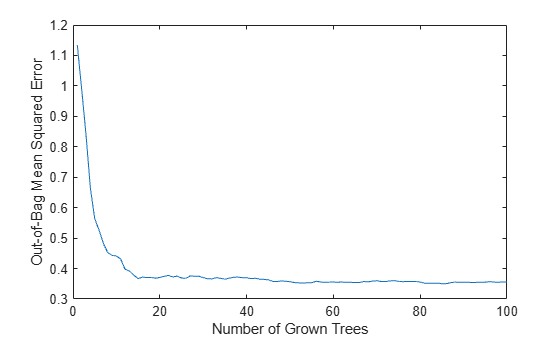```figure bar(b5v.OOBPermutedPredictorDeltaError) xlabel('Feature Index') ylabel('Out-of-Bag Feature Importance')```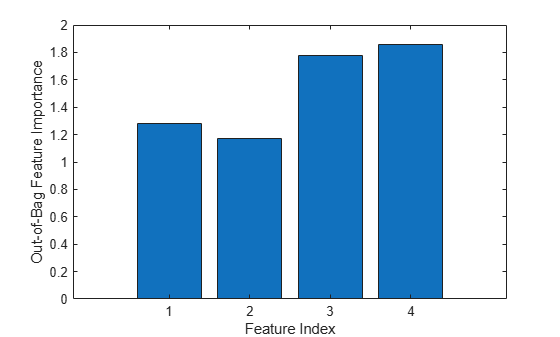These four most powerful features give the same MSE as the full set, and the ensemble trained on the reduced set ranks these features similarly to each other. If you remove features 1 and 2 from the reduced set, then the predictive power of the algorithm might not decrease significantly.

### Finding Outliers

To find outliers in the training data, compute the proximity matrix using `fillProximities`.

`b5v = fillProximities(b5v);`

The method normalizes this measure by subtracting the mean outlier measure for the entire sample. Then it takes the magnitude of this difference and divides the result by the median absolute deviation for the entire sample.

```figure histogram(b5v.OutlierMeasure) xlabel('Outlier Measure') ylabel('Number of Observations')```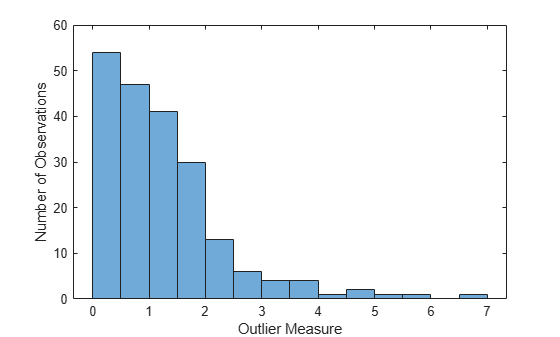### Discovering Clusters in the Data

By applying multidimensional scaling to the computed matrix of proximities, you can inspect the structure of the input data and look for possible clusters of observations. The `mdsProx` method returns scaled coordinates and eigenvalues for the computed proximity matrix. If you run it with the `Colors` name-value-pair argument, then this method creates a scatter plot of two scaled coordinates.

```figure(8) [~,e] = mdsProx(b5v,'Colors','K'); xlabel('First Scaled Coordinate') ylabel('Second Scaled Coordinate')```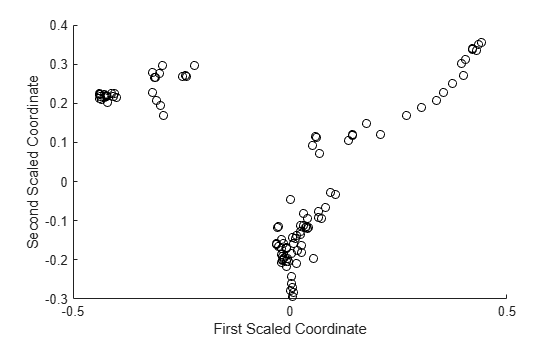Assess the relative importance of the scaled axes by plotting the first 20 eigenvalues.

```figure bar(e(1:20)) xlabel('Scaled Coordinate Index') ylabel('Eigenvalue')```### Saving the Ensemble Configuration for Future Use

To use the trained ensemble for predicting the response on unseen data, store the ensemble to disk and retrieve it later. If you do not want to compute predictions for out-of-bag data or reuse training data in any other way, there is no need to store the ensemble object itself. Saving the compact version of the ensemble is enough in this case. Extract the compact object from the ensemble.

`c = compact(b5v)`
```c = CompactTreeBagger Ensemble with 100 bagged decision trees: Method: regression NumPredictors: 4 Properties, Methods ```

You can save the resulting `CompactTreeBagger` model in a `*``.mat` file.